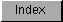```
The KBD_READMATRIX command returns the current state of every key in the
key matrix (up = 0,  down = 1). You provide a data area that is at least
large enough to hold one bit per key, approximately 16 bytes.  The
keyboard layout for the A500, A2000 and A3000 is shown in the figure
below, indicating the raw numeric value that each key transmits when it is
pressed.  This value is the numeric position that the key occupies in the
key matrix.

Figure 7-1 - Keyboard Layout

The following example will read the key matrix and display the up-down
state of all of the elements in the matrix in a table.  Reading the column
header and then the row number as a hex number gives you the raw key code.

In addition to the matrix data returned in io_Data, io_Actual
returns the number of bytes filled in io_Data with key matrix data,
i.e., the minimum of the supplied length and the internal key matrix
size.

Value of io_Length.
-------------------
A value of 13 in the io_Length field will be sufficient for most
keyboards; extended keyboards will require a larger number.  However,
you must always set this field to 13 for V34 and earlier versions of
Kickstart.

To find the status of a particular key - for example, to find out if the
F2 key is down - you find the bit that specifies the current state by
dividing the key matrix value by 8. Since hex 51 = 81, this indicates that
the bit is in byte number 10 of the matrix. Then take the same number
(decimal 81) and use modulo 8 to determine which bit position within that
byte represents the state of the key. This yields a value of 1. So, by
reading bit position 1 of byte number 10, you determine the status of the
function key F2.

```